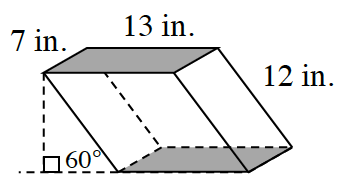### Home > INT2 > Chapter 11 > Lesson 11.2.1 > Problem11-46

11-46.Elliot has a modern fish tank that is in the shape of an oblique prism, shown at right.

1. If the slant of the prism makes a $60°$ angle with the flat surface on which the prism is placed, what is the volume of water the tank can hold? Assume that each base is a rectangle.

Multiply the area of the base times the height. You can find the height by using the special properties of a $30°$ - $60°$ - $90°$ right triangle.

2. What is the volume of Elliot’s tank in gallons if one cubic foot of water equals $7.48$ gallons? Show your steps and work.

$945.7\;\text{in}^{3}\cdot\frac{(1\;\text{foot})^{3}}{(12\;\text{in})^{3}}= .547\;\text{ft}^{3}$

$.547\;\text{ft}^{3}\cdot\frac{7.48\; \text{gallons}}{(1\;\text{foot})^{3}}=...$

$≈ 4.1$ gallons